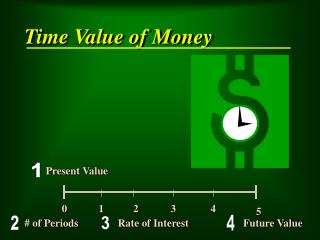DownloadDownload PresentationTime Value of Money

# Time Value of Money

Download Presentation## Time Value of Money

- - - - - - - - - - - - - - - - - - - - - - - - - - - E N D - - - - - - - - - - - - - - - - - - - - - - - - - - -
##### Presentation Transcript

1. 1 Present Value 0 1 2 3 4 5 4 # of Periods Rate of Interest Future Value 2 3 Time Value of Money

2. Computing Simple Interest I = P x R x T Interest Principal Annual Rate Period of Time the Note of Note of Interest is Outstanding in Years Compound Interest Interest is earned on both the initial principal plus any interest previously earned

3. n F = P x (1 + r) n or F = P x FVF n,r n The Future Value of a Single Lump Sum Amount... … is described as the amount that money invested today will be worth at a future point in time

4. Future Value Calculations are used for planning purposes Present Value Calculations are used to measure the value of business transactions in U.S. Dollars • Notes Receivable • Notes Payable • Leases • Amortization of Premiums • Amortization of Discounts • Pensions • Long-term Assets • Sinking Funds • Business Combinations • Disclosures • Installment Contracts

5. Major Time Value Concepts: • Future Value of a Single, Lump Sum Amount • Future Value of an Ordinary Annuity • Future Value of an Annuity Due • Present Value of a Single, Lump Sum Amount • Present Value of an Ordinary Annuity • Present Value of an Annuity Due • Deferred Annuities

6. Calculating the Present Value of an Annuity Due 1) Find the present value of an ordinary annuity factor (PVF-OA @ n=N-1, r=%) for n-1 periods 2) Add “1” to that factor to get the “PVF-AD” factor 3) Multiply the periodic “amount” times this PVF-AD to get the present value of payments

7. Calculating the Present Value of a Deferred Annuity • Calculate the deferred annuity factor • (a) Find the “factor” assuming payment started the first year (assuming no deferral) • (b) Find the “factor” relating the period of time when payment is not made • (c) Subtract (b) from (a) • Use this new calculation as the factor in your calculation